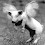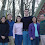### Testing for divisibility

Sometimes it's useful to find out if a large number is divisible by a small number without leaving any remainder. For example, I could be delivering a presentation to a group of 27 engineers and I want to divide them into equal-sized teams. I know that I can divide them into 3 teams of 9, because 27 is divisible by 3. If there were 28 engineers, the teams would be different sizes. Then I might divide them into 4 teams of 7 instead, because 28 is divisible by 4. (If there were 29 engineers, it would really irritate me because 29 is a prime number and I'm neurotic that way.)

How do you quickly test for divisibility by various numbers?

You probably already know that any number that ends in 0, 2, 4, 6, or 8 is an even number, meaning that it is divisible by 2.

To find out if a number is divisible by 3, add all its digits together. If the sum is divisible by 3, then the original number is divisible by 3. For example, 13986 is divisible by 3 because 1+3+9+8+6 = 27, and 27 is divisible by 3.

To test whether a number is divisible by 4, check whether the last two digits are divisible by 4. For example, 83924 is divisible by 4 because 24 is divisible by 4. (If you're not sure whether a larger number like 84 or 88 is divisible by 4, simply divide it by 2; if you end up with an even number, then it is divisible by 4.)

It's easy to find out if a number is divisible by 5. If the last digit is a 5 or a 0, the number is divisible by 5.

How about 6? This takes two steps. First, check that it is even (ends in 0, 2, 4, 6, or 8). If so, then check if it is divisible by 3 using the method described above. If the number passes both tests, then it is divisible by 6.

Testing for divisibility by 7 is a bit more complicated, but you can still do it in your head. Remove the last digit, double it, and subtract it from the rest of the original number. Repeat this step if necessary until you know whether the result is divisible by 7, in which case the original number is divisible by 7. Example: To test 7308 for divisibility by 7, take the last digit 8 and double it to get 16 then subtract from 730 to get 714. At this point you might notice that 714 is divisible by 7 because it is 700 + 14 and both of those numbers are divisible by 7. But just in case you didn't notice that, repeat the process; take the last digit 4 and double it to get 8 and subtract from 71 to get 63. Since 63 is divisible by 7, the original number 7308 is divisible by 7.

To check if a number is divisible by 8, look at the last three digits. If they form a number that is divisible by 8, then the entire number is divisible by 8. For example, 238272 is divisible by 8 because 272 is divisible by 8. (To check whether a larger number such as 272 is divisible by 8, divide it by 2 twice and see if you still end up with an even number.)

To check if a number is divisible by 9, add up all its digits and see whether the sum is divisible by 9. For example, 884916 is divisible by 9 because 8+8+4+9+1+6 = 36, which is 9 x 4.

To check if a number is divisible by 10, just check that the last digit is 0. That one's the easiest of all!Deb said…
*Insert Blank Expression Here*Sassan Sanei said…
Okay, let me give you an example of why this would be useful.

Suppose you have an image that you want to post on your blog. The original picture is 2336 x 3504 pixels - too huge for a web site. You want to reduce it down to about 400 x 600 or so.

You also want to preserve as much of the original image quality as possible, even though you are going to a lower resolution. One way to do that is to reduce the size by a fraction of a whole number, such as 1/5 or 1/6 (but not 1/5.6789) - see note 1 for why.

The problem is that 2336 is not divisible by 6. If you divide 2336 by 6, you get 389.3, not a whole number.

So you look for the next lower number down from 2336 that is divisible by 6. You know from reading my blog that this would be an even number whose digits all add up to a number that is divisible by 3. That number is 2334 (it's an even number, and 2+3+3+4=12, which is divisible by 3; therefore, 2334 is divisible by 6). Now you can crop your picture to 2334 pixels before scaling down by a factor of 6.

But wait! The other dimension is still 3504. But that's okay, because using the same test, we find that 3504 is divisible by 6 - see note 2 for a caution. So cropping the picture to 2334 x 3504, then scaling by a factor of 6 (down to 389 x 584), brings us close the 400 x 600 pixel picture that we wanted.

The picture quality will be better than if we just blindly scaled down to 400 x 600. That's just one way that testing for divisibility is useful.

Notes:

1. The advantage of scaling by a factor of 1/6 instead of something like 1/5.84 is that every pixel in the original image is represented in only one pixel of the new image, so that we are not smearing image data from one source pixel across two destination pixels.

2. Dropping two pixels from one dimension only caused a slight change in the aspect ratio. The error is less than 0.1%, so I suggest living with it. A purist might insist on cropping to 2328 x 3492 pixels, then scaling to 388 x 582, in order to preserve the exact aspect ratio.Deb said…
Okay you win. When I leave Oklahoma and my IQ rises to normal levels again, I'll look at your math entries line by line until I understand everything you said.

Howsat?Deb said…
I'm home now. I am going to spend the entire week studying this stuff until I understand it better than you do.

I love a challenge.hunghuynh said…
very good
I love a challenge.Anonymous said…
If you wish to know methods to check divisibility by 7 that are acurate and almost as fast to apply as the test of divisibility by 9, visit the site:

www.7and13divisibility.com

Especially the method that works with the inclusion of digits:

Example:

n = 861

(2) 861 (4)

S = 2 + 8 + 6 + 1 + 4
S = 21
If 7 divides S then 7 divides n